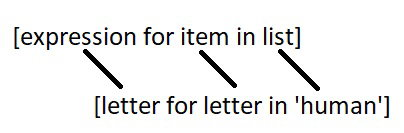# Python List Comprehension

## List Comprehension vs For Loop in Python

Suppose, we want to separate the letters of the word `human` and add the letters as items of a list. The first thing that comes in mind would be using for loop.

#### Example 1: Iterating through a string Using for Loop

``````h_letters = []

for letter in 'human':
h_letters.append(letter)

print(h_letters)``````

When we run the program, the output will be:

`['h', 'u', 'm', 'a', 'n']`

However, Python has an easier way to solve this issue using List Comprehension. List comprehension is an elegant way to define and create lists based on existing lists.

Let’s see how the above program can be written using list comprehensions.

### Example 2: Iterating through a string Using List Comprehension

``````h_letters = [ letter for letter in 'human' ]
print( h_letters)``````

When we run the program, the output will be:

`['h', 'u', 'm', 'a', 'n']`

In the above example, a new list is assigned to variable h_letters, and list contains the items of the iterable string 'human'. We call `print()` function to receive the output.

### Syntax of List Comprehension

`[expression for item in list]`We can now identify where list comprehensions are used.

If you noticed, `human` is a string, not a list. This is the power of list comprehension. It can identify when it receives a string or a tuple and work on it like a list.

You can do that using loops. However, not every loop can be rewritten as list comprehension. But as you learn and get comfortable with list comprehensions, you will find yourself replacing more and more loops with this elegant syntax.

## List Comprehensions vs Lambda functions

List comprehensions aren’t the only way to work on lists. Various built-in functions and lambda functions can create and modify lists in less lines of code.

#### Example 3: Using Lambda functions inside List

``````letters = list(map(lambda x: x, 'human'))
print(letters)``````

When we run the program, the output will be

`['h','u','m','a','n']`

However, list comprehensions are usually more human readable than lambda functions. It is easier to understand what the programmer was trying to accomplish when list comprehensions are used.

## Conditionals in List Comprehension

List comprehensions can utilize conditional statement to modify existing list (or other tuples). We will create list that uses mathematical operators, integers, and range().

#### Example 4: Using if with List Comprehension

``````number_list = [ x for x in range(20) if x % 2 == 0]
print(number_list)``````

When we run the above program, the output will be:

`[0, 2, 4, 6, 8, 10, 12, 14, 16, 18]`

The list ,number_list, will be populated by the items in range from 0-19 if the item's value is divisible by 2.

#### Example 5: Nested IF with List Comprehension

``````num_list = [y for y in range(100) if y % 2 == 0 if y % 5 == 0]
print(num_list)``````

When we run the above program, the output will be:

`[0, 10, 20, 30, 40, 50, 60, 70, 80, 90]`

Here, list comprehension checks:

1. Is y divisible by 2 or not?
2. Is y divisible by 5 or not?

If y satisfies both conditions, y is appended to num_list.

#### Example 6: if...else With List Comprehension

``````obj = ["Even" if i%2==0 else "Odd" for i in range(10)]
print(obj)``````

When we run the above program, the output will be:

`['Even', 'Odd', 'Even', 'Odd', 'Even', 'Odd', 'Even', 'Odd', 'Even', 'Odd']`

Here, list comprehension will check the 10 numbers from 0 to 9. If i is divisible by 2, then `Even` is appended to the obj list. If not, `Odd` is appended.

## Nested Loops in List Comprehension

Suppose, we need to compute the transpose of a matrix that requires nested for loop. Let’s see how it is done using normal for loop first.

#### Example 7: Transpose of Matrix using Nested Loops

``````transposed = []
matrix = [[1, 2, 3, 4], [4, 5, 6, 8]]

for i in range(len(matrix)):
transposed_row = []

for row in matrix:
transposed_row.append(row[i])
transposed.append(transposed_row)

print(transposed)``````

Output

```[[1, 4], [2, 5], [3, 6], [4, 8]]
```

The above code use two for loops to find transpose of the matrix.

We can also perform nested iteration inside a list comprehension. In this section, we will find transpose of a matrix using nested loop inside list comprehension.

#### Example 8: Transpose of a Matrix using List Comprehension

``````matrix = [[1, 2], [3,4], [5,6], [7,8]]
transpose = [[row[i] for row in matrix] for i in range(2)]
print (transpose)``````

When we run the above program, the output will be:

`[[1, 3, 5, 7], [2, 4, 6, 8]]`

In above program, we have a variable matrix which have `4` rows and `2` columns.We need to find transpose of the matrix. For that, we used list comprehension.

**Note: The nested loops in list comprehension don’t work like normal nested loops. In the above program, `for i in range(2)` is executed before `row[i] for row in matrix`. Hence at first, a value is assigned to i then item directed by `row[i]` is appended in the transpose variable.

## Key Points to Remember

• List comprehension is an elegant way to define and create lists based on existing lists.
• List comprehension is generally more compact and faster than normal functions and loops for creating list.
• However, we should avoid writing very long list comprehensions in one line to ensure that code is user-friendly.
• Remember, every list comprehension can be rewritten in for loop, but every for loop can’t be rewritten in the form of list comprehension.